GCSE Maths Statistics

# Statistics

Here you will find all the GCSE statistics revision guides you need - over 40 in total. As for each of our GCSE maths revision topics, we've included step by step guides, detailed examples, practice questions, exam questions and statistics worksheets! All GCSE questions are based on actual Edexcel, AQA and OCR exam questions with mark scheme and model answers provided.

Filter by:

Representing Data

Statistics

Frequency Tables

Histograms

Types of Sampling

Cumulative Frequency

Mean Median Mode

Types of Data

Range

Show all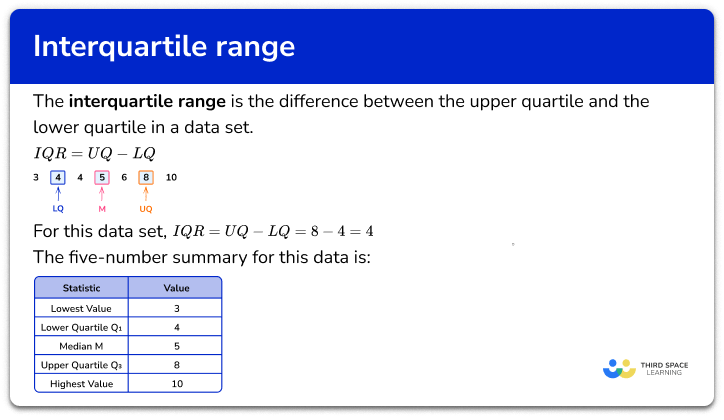Interquartile range

Step by step guide, detailed examples, practice questions, exam questions and interquartile range worksheets.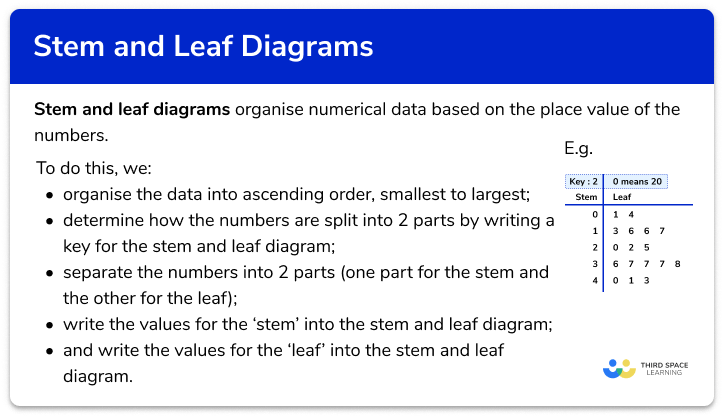Stem and leaf diagram

Step by step guide, detailed examples, practice questions, exam questions and stem and leaf diagram worksheets.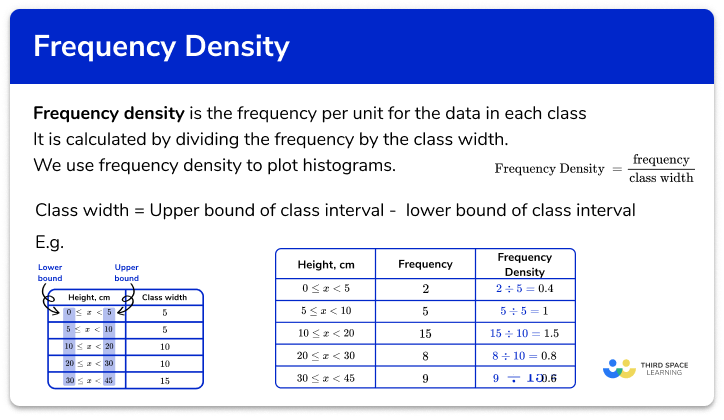Frequency density

Step by step guide, detailed examples, practice questions, exam questions and frequency density worksheets.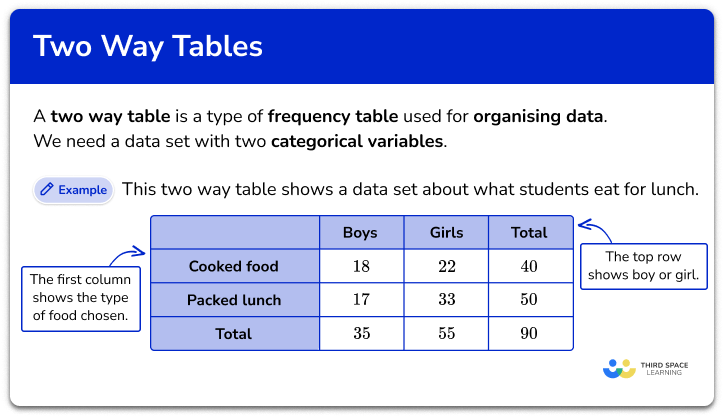Two way tables

Step by step guide, detailed examples, practice questions, exam questions and two way tables worksheets.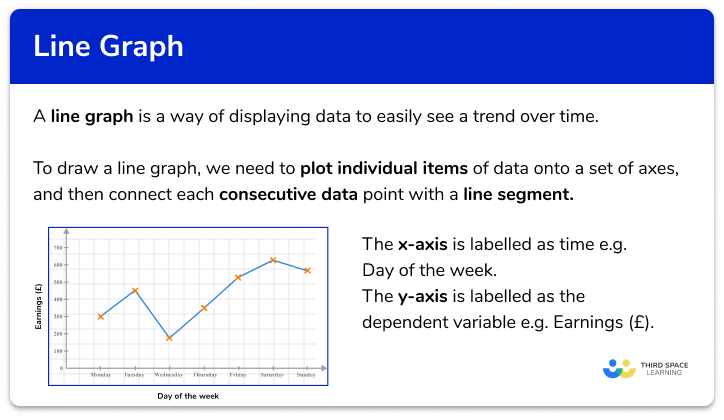Line graph

Step by step guide, detailed examples, practice questions, exam questions and line graph worksheets.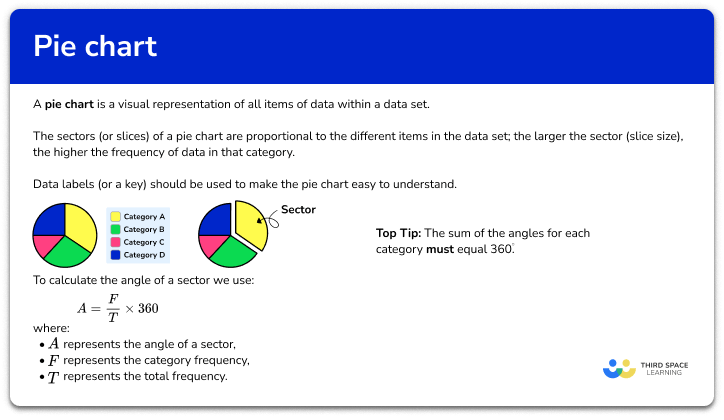Pie Chart

Step by step guide, detailed examples, practice questions, exam questions and pie chart worksheets.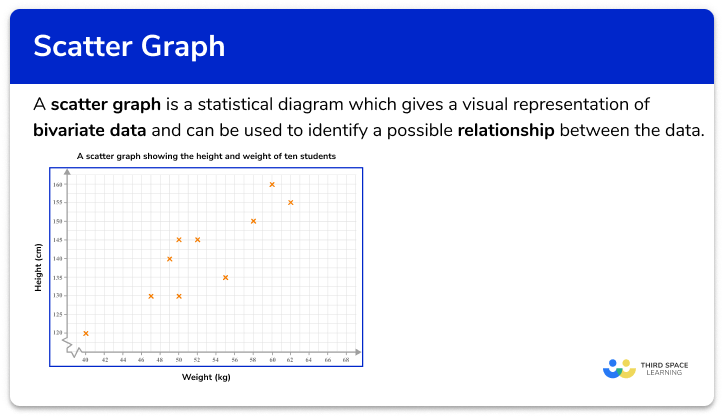Scatter Graphs

Step by step guide, detailed examples, practice questions, exam questions and scatter graphs worksheets.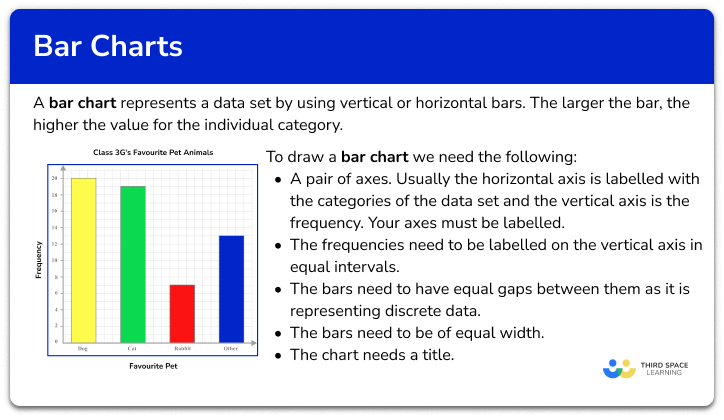Bar Chart

Step by step guide, detailed examples, practice questions, exam questions and bar chart worksheets.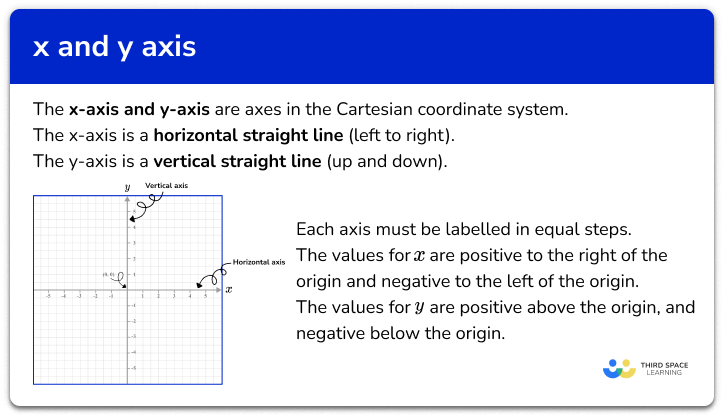x and y axis

Step by step guide, detailed examples, practice questions, exam questions and x and y axis worksheets.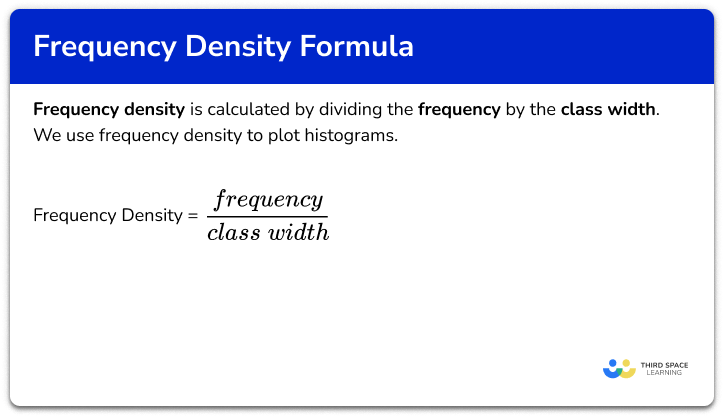Frequency density formula

Step by step guide, detailed examples, practice questions, exam questions and frequency density formula worksheets.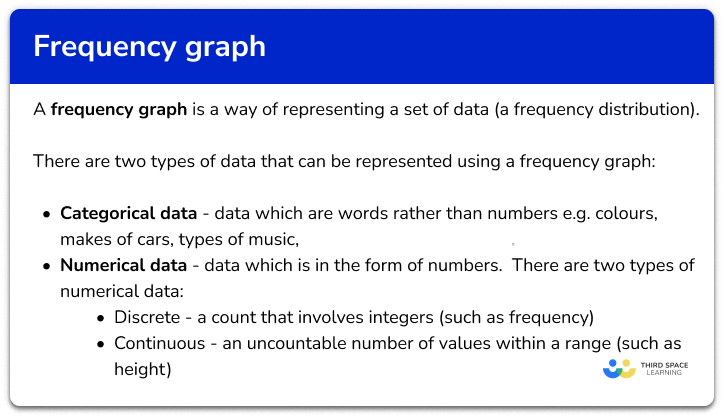Frequency graph

Step by step guide, detailed examples, practice questions, exam questions and frequency graph worksheets.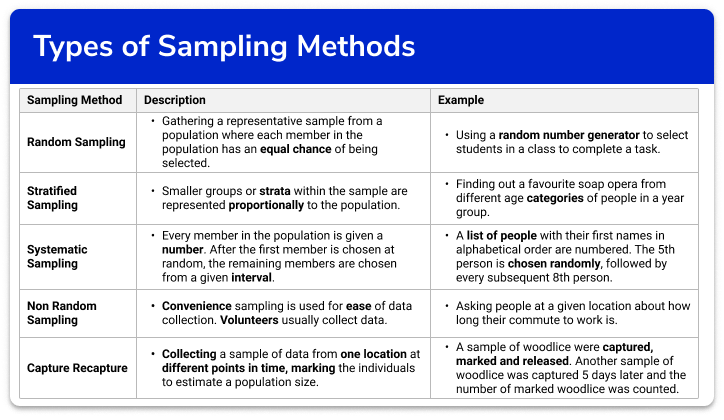Types of sampling methods

Step by step guide, detailed examples, practice questions, exam questions and sampling methods worksheets.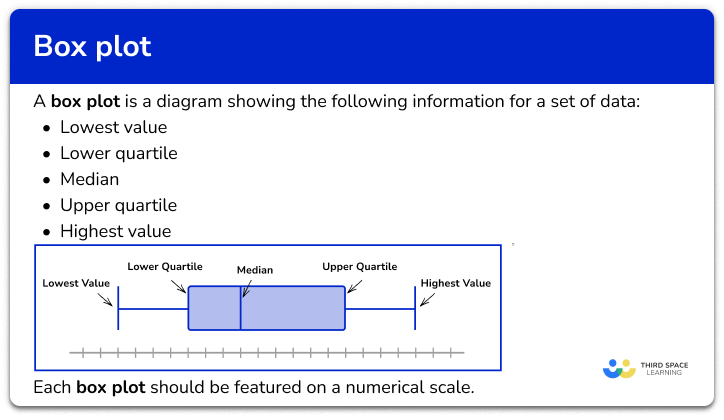Box Plot

Step by step guide, detailed examples, practice questions, exam questions and box plot worksheets.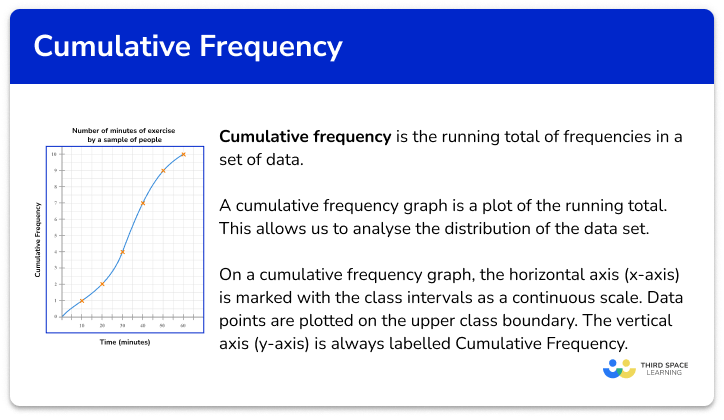Cumulative frequency

Step by step guide, detailed examples, practice questions, exam questions and cumulative frequency worksheets.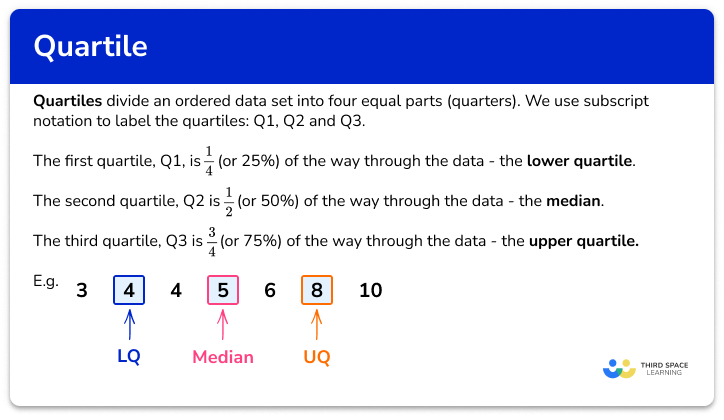Quartile

Step by step guide, detailed examples, practice questions, exam questions and quartile worksheets.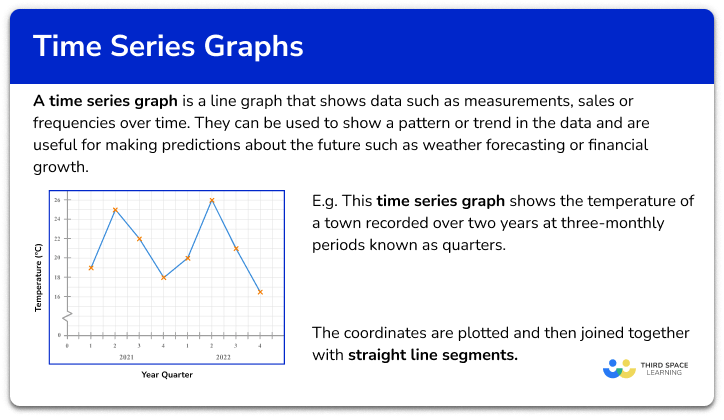Time series graph

Step by step guide, detailed examples, practice questions, exam questions and time series graph worksheets.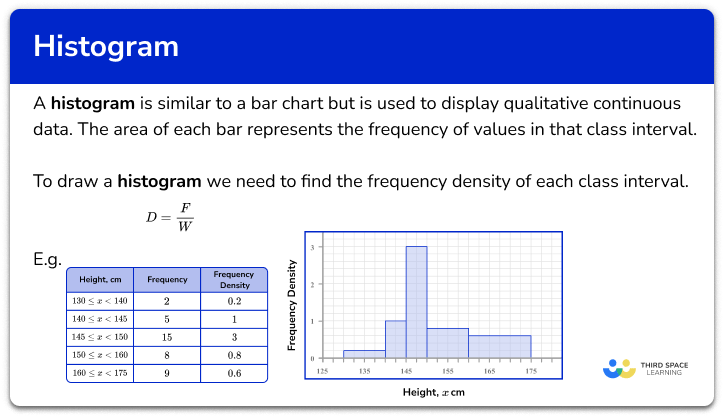Histogram

Step by step guide, detailed examples, practice questions, exam questions and histogram worksheets.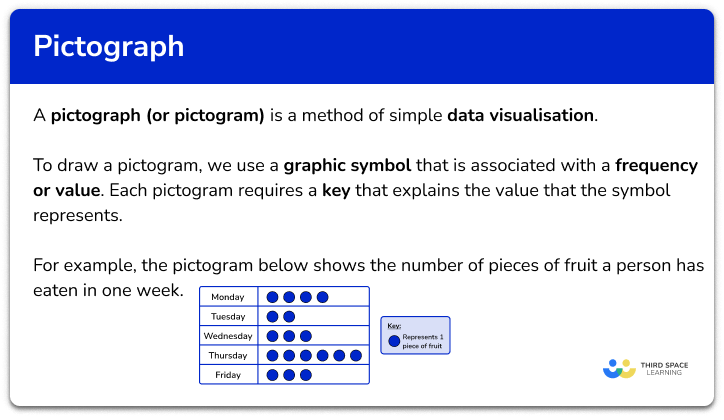Pictograph

Step by step guide, detailed examples, practice questions, exam questions and pictograph worksheets.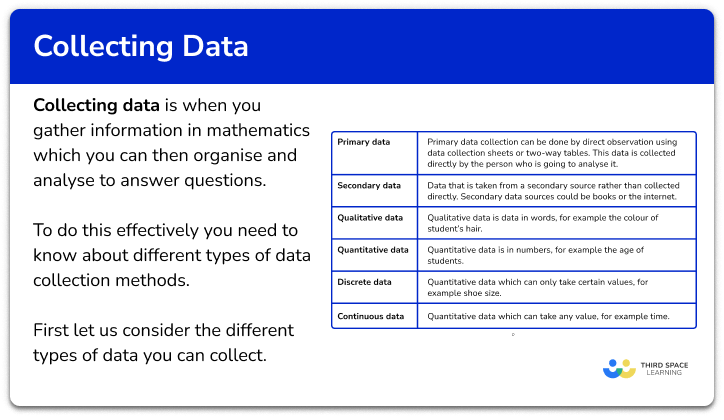Collecting data

Step by step guide, detailed examples, practice questions, exam questions and collecting data worksheets.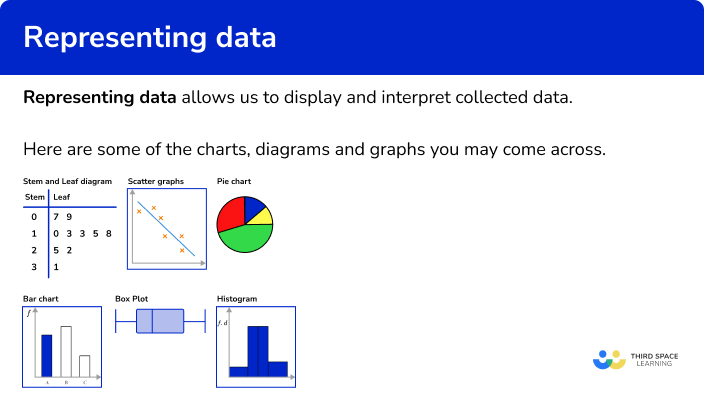Representing data

Step by step guide, detailed examples, practice questions, exam questions and representing data worksheets.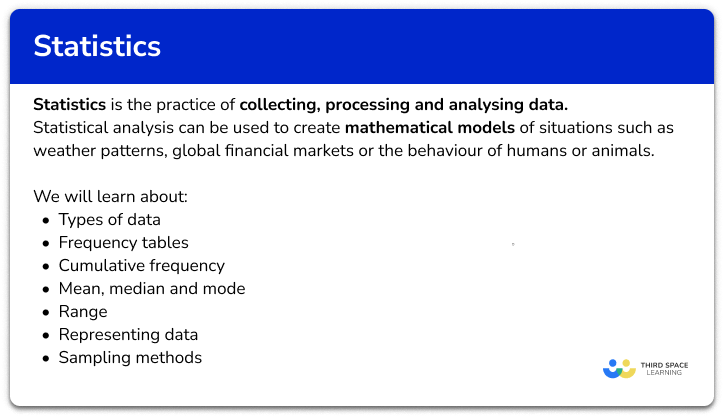Statistics – Maths GCSE

Step by step guide, detailed examples, practice questions, exam questions and statistics maths worksheets.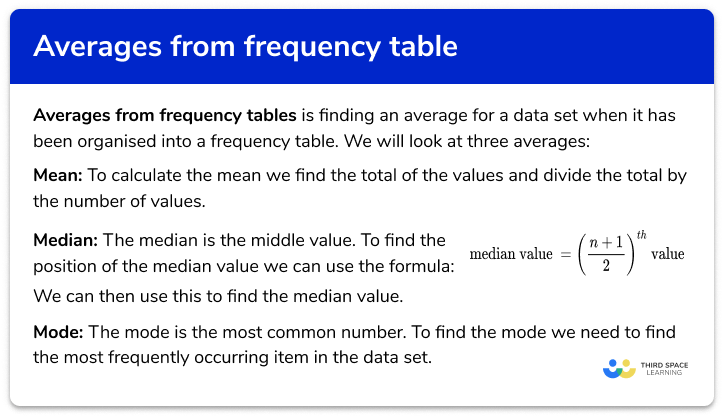Averages from frequency tables

Step by step guide, detailed examples, practice questions, exam questions and averages from frequency tables worksheets.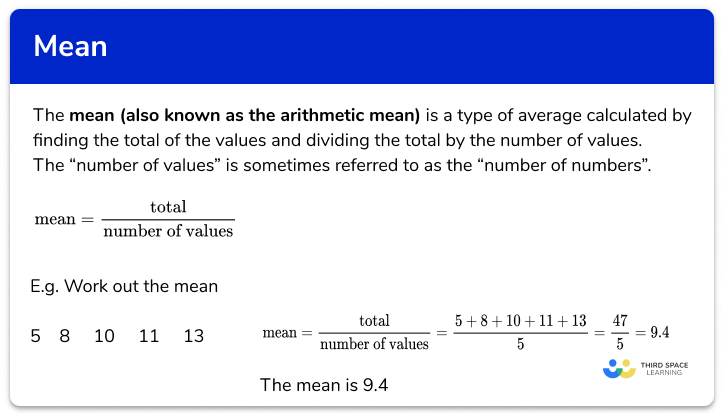Mean

Step by step guide, detailed examples, practice questions, exam questions and mean maths worksheets.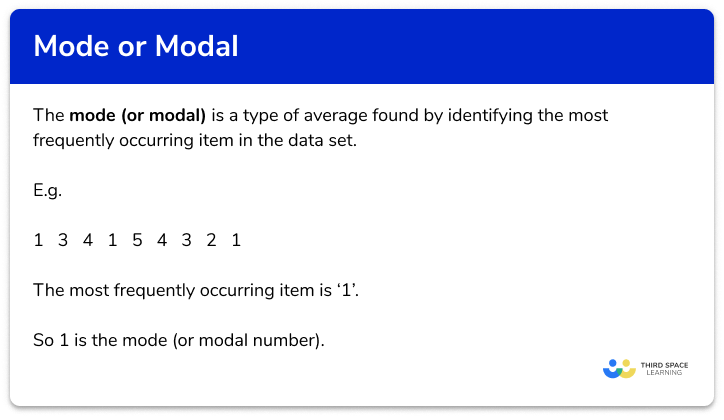Mode or Modal

Step by step guide, detailed examples, practice questions, exam questions and mode maths or modal maths worksheets.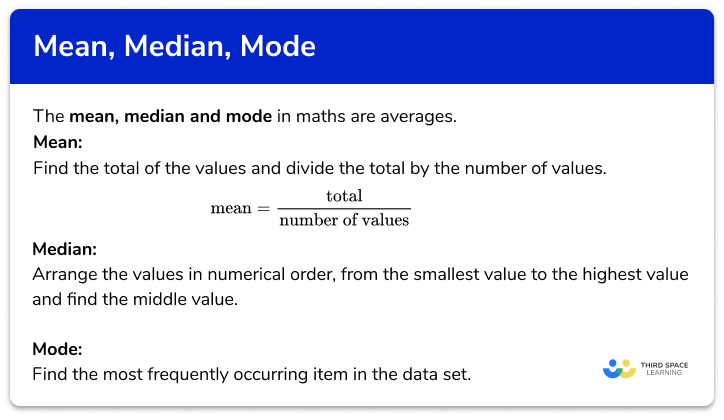Mean, median, mode

Step by step guide, detailed examples, practice questions, exam questions and mean median mode worksheets.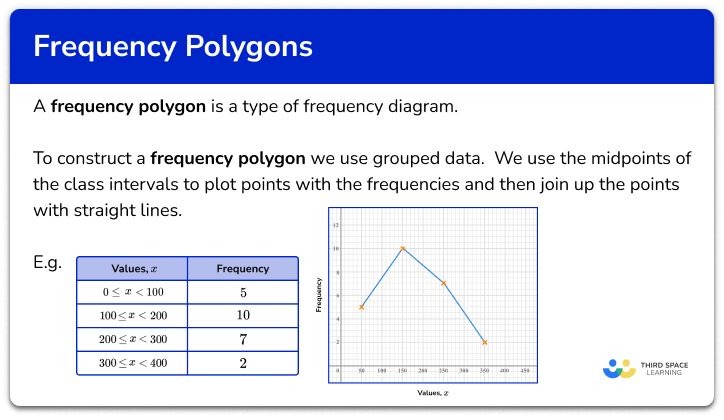Frequency polygon

Step by step guide, detailed examples, practice questions, exam questions and frequency polygon worksheets.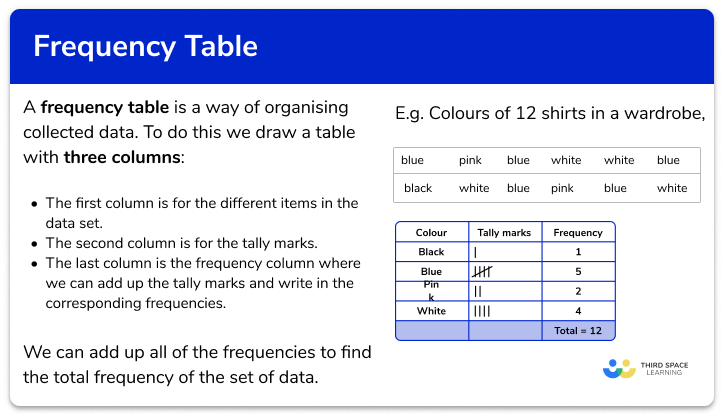Frequency table

Step by step guide, detailed examples, practice questions, exam questions and frequency table worksheets.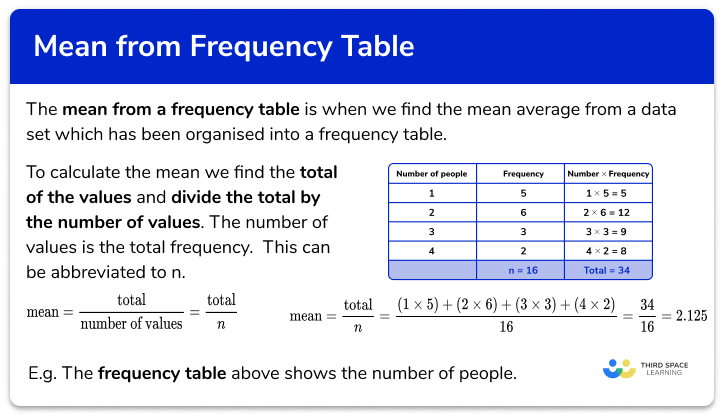Mean from a frequency table

Step by step guide, detailed examples, practice questions, exam questions and mean from a frequency table worksheets.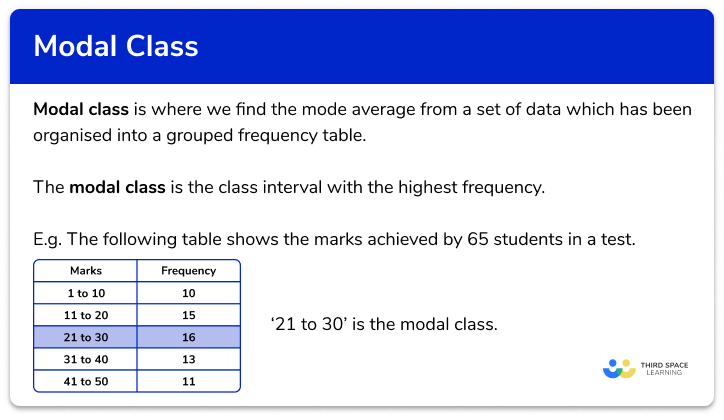Modal class

Step by step guide, detailed examples, practice questions, exam questions and modal class worksheets.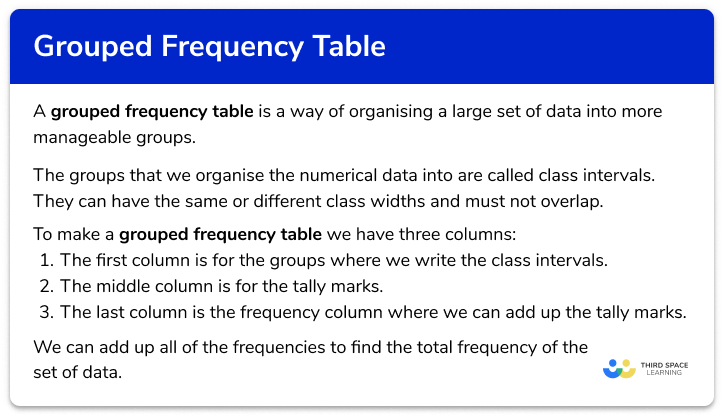Grouped frequency table

Step by step guide, detailed examples, practice questions, exam questions and grouped frequency table worksheets.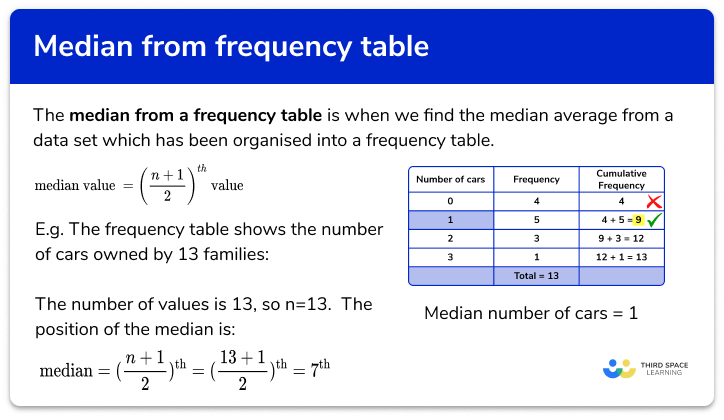Median from a frequency table

Step by step guide, detailed examples, practice questions, exam questions and median from a frequency table worksheets.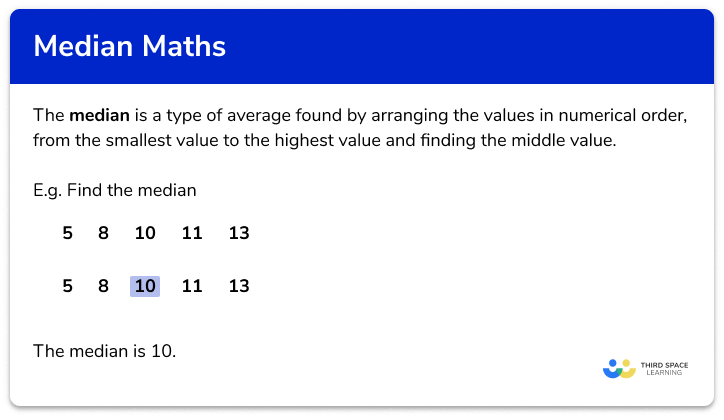Median

Step by step guide, detailed examples, practice questions, exam questions and median maths worksheets.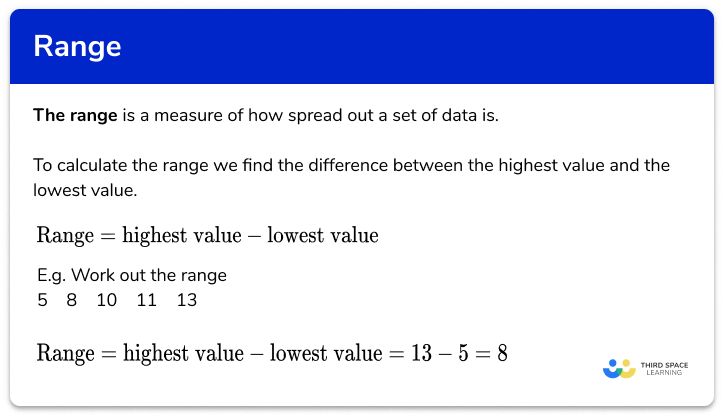Range

Step by step guide, detailed examples, practice questions, exam questions and range maths worksheets.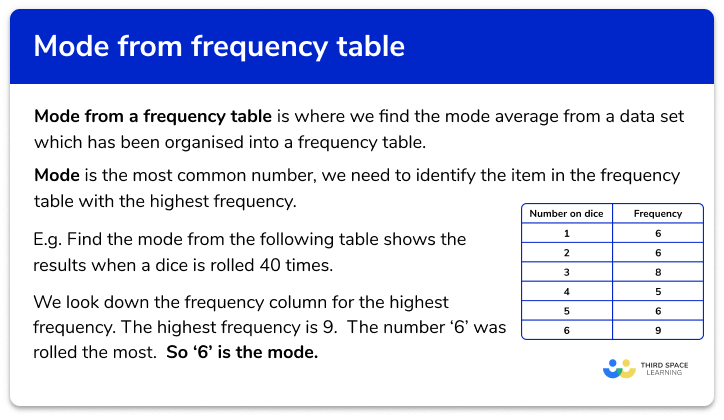Mode from a frequency table

Step by step guide, detailed examples, practice questions, exam questions and mode from a frequency table worksheets.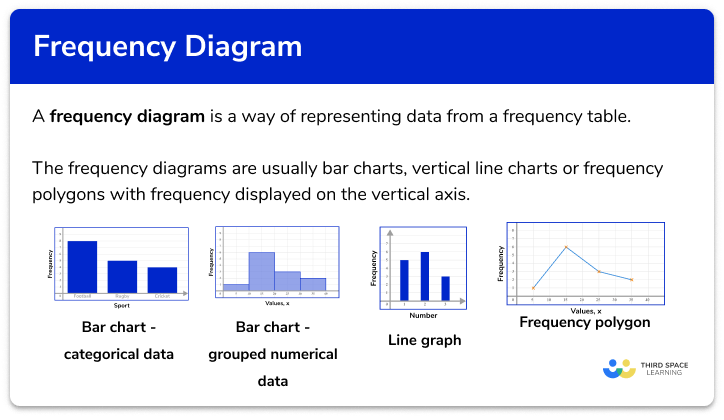Frequency diagram

Step by step guide, detailed examples, practice questions, exam questions and frequency diagram worksheets.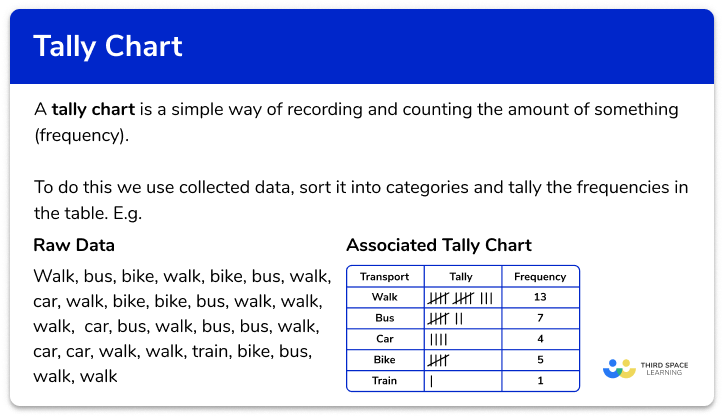Tally chart

Step by step guide, detailed examples, practice questions, exam questions and tally chart worksheets.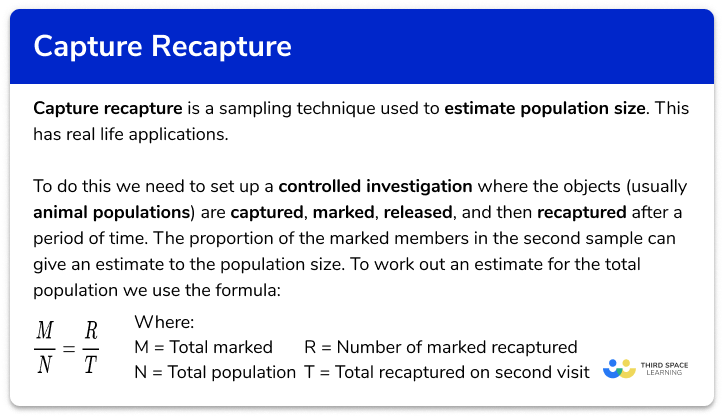Capture recapture

Step by step guide, detailed examples, practice questions, exam questions and capture recapture worksheets.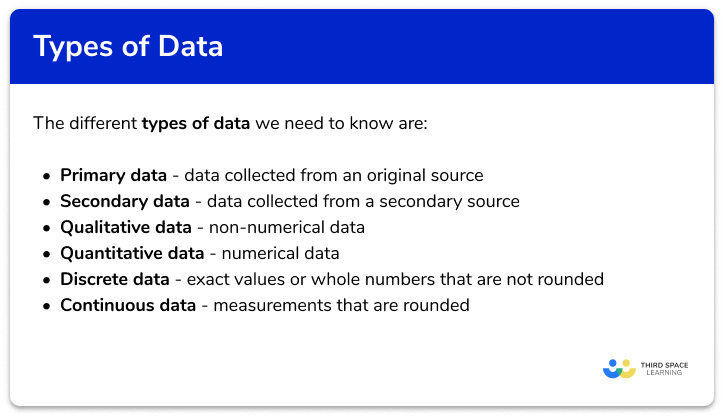Types of data

Step by step guide, detailed examples, practice questions, exam questions and types of data worksheets.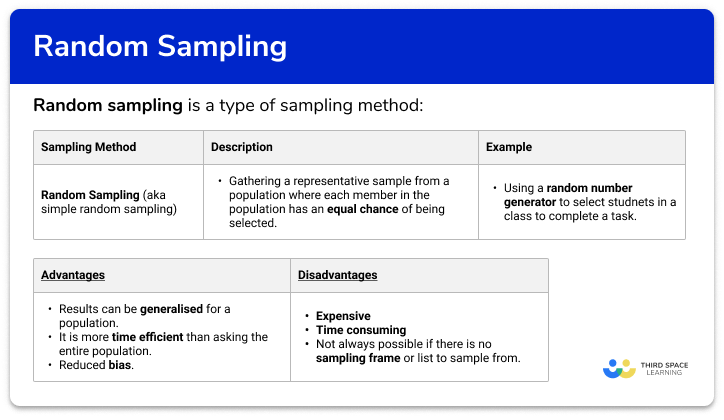Random sampling

Step by step guide, detailed examples, practice questions, exam questions and random sampling worksheets.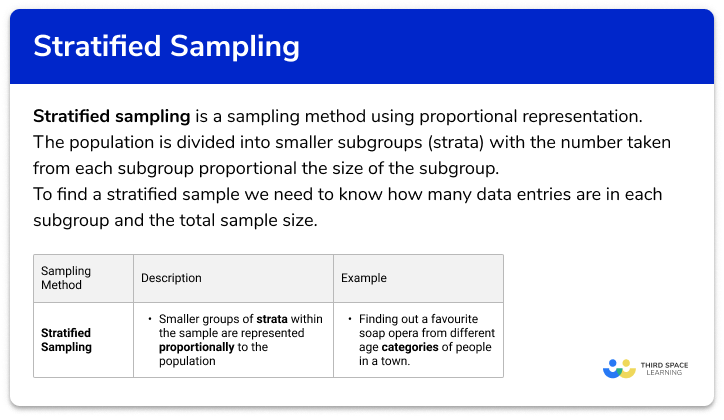Stratified sampling

Step by step guide, detailed examples, practice questions, exam questions and stratified sampling worksheets.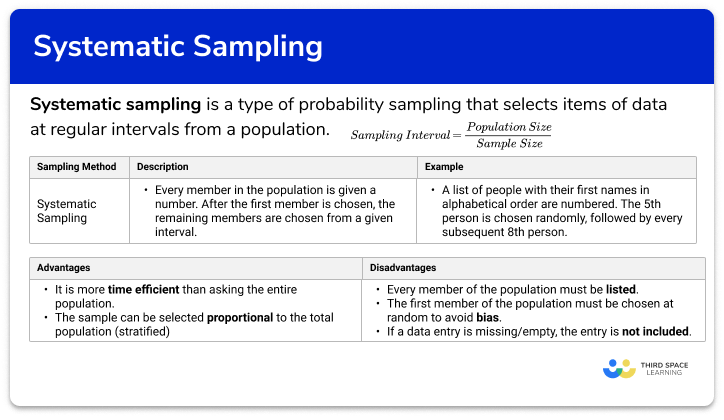Systematic sampling

Step by step guide, detailed examples, practice questions, exam questions and systematic sampling worksheets.

One to one maths interventions built for KS4 success

Weekly online one to one GCSE maths revision lessons now available